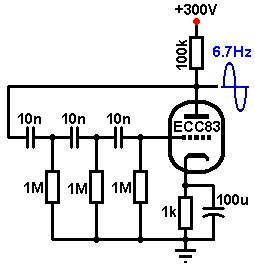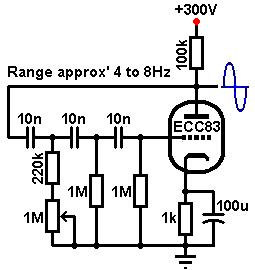# The Valve Wizard

How to design valve guitar amplifiers!

The Phase-Shift Oscillator for Tremolo

Tremolo, or slow amplitude modulation, is a simple and popular effect used to add texture and character to the guitar sound, in the same way that vibrato (pitch modulation) is used by vocalists, violinists and other string players to add body to an otherwise unvarying note. Many popular amps have included this effect, notably the Marshall 18W and Fender Vibroverb / Vibrochamp etc. models. (Note that despite the misleading names, these amps employed tremolo and not vibrato, which requires much greater circuit complexity.) Nearly all use the same basic tremolo circuit. The effect requires a low frequency oscillator (LFO), which produces a wave (usually a sine wave) of around 0.5 to 10Hz- the "trem' signal". This is then mixed with the audio signal in some way, so that its amplitude (volume) increases and decreases in sympathy with the trem' signal. The effect usually has controls to vary both the frequency (speed) and amplitude (depth / intensity) of the tremolo, allowing the effect to be used quite subtly or very vividly.
It is worth mentioning, that of all the stages in an amplifier the trem' oscillaor is the most sensitive to valve ageing. If you have a very weak sounding tremolo it can usually be ammended by plugging in a new valve.

Most oscillators works by means of positive feedback around one or more gain stages. Provided the feedback signal is in phase with the input signal, and the total loop gain is unity or greater, the circuit will oscillate at a constant frequency indefinitely. Note that the trem' signal does not have to be a sine wave; more elaborate circuits allow the user to vary the waveform of the trem' signal. Square and triangular waves give a well defined tremolo, or even a stutter effect. However, a sine wave is easy to create and has a natural sound. For a more elaborate trem' it's probably easier to just add an effects loop to the amp and use an off-board, dedicated trem' unit.Literally dozens of oscillator circuits can be found in textbooks, but by far the most popular for guitar is the "phase-shift oscillator". This comprises a single amplifier stage (itself providing 180 degrees of phase shift) and a feedback network of capacitors and resistors (providing a further 180 degrees phase shift, = 360 degrees total shift). The feedback network usually consists of three CR filters- C1/R1, C2/R2, C3/R3. Ideally, each filter provides 60 degree of phase shift at one particular frequency, and this is the frequency at which the circuit will oscillate. A four stage CR network could also be used, each filter providing 45 degrees of shift.
The total loop gain must be unity or greater, so any loss in the feedback network must be compensated for by the gain of the amplifier. Without going into the maths of the network (which would require either some lengthy equations or vector analysis) it can be shown that the gain of a three-stage CR network, at a frequency where each filter provides 60 degrees shift, is -0.034. Since 1 / -0.034 = -29, this is the minimum necessary gain we need from the amplifier to sustain oscillations. (In a four stage network a gain of only -18.4 would be required.) If the amplifier has a gain of exactly this value it will produce a near perfect sine wave. However, the stage would be highly unstable and would quickly stop working as the valve aged and gain fell, and would require continual adjustment- especially if we want to vary the frequency. Instead, the amplifier is set up for a gain level much greater than -29. The drawback is that the output signal will be somewhat distorted as high harmonics are introduced. Stray capacitance will eliminate some, but in any case it is of no great concern for something as crude as a trem' oscillator.

Immediately we know we require either a high gain triode, or a pentode. The ECC81 is a favourite choice for oscillator circuits, and in most cases can be directly substituted for an ECC83. The following example uses the more common ECC83, and the HT is 300V. Note that the HT does not have to be particularly well filtered since we are not amplifying audio signal currents, and the circuit will even work at supply voltages as low as 100V.

A fairly large anode resistor is in order, to maximize gain and output swing, and 100k is typical. For the purest waveform the stage should be biased for maximum linear swing, although it is not critical. To keep the output impedance as low as possible, and to maximize gain, the cathode should be fully decoupled well below the oscillation frequency. A 100uF to 220uF capacitor will usually suffice (even larger values are available, but tend to be unreliable as well as unnecessary). With the usual 100k anode resistor we can make an estimation of gain as:
Av = (mu * Ra) / (Ra + ra)
Av = (100 * 100000) / (100000 + 55000)
= 64.5
This is plenty of gain, although it does not yet take account of the loading effect of the feedback network or any other external circuit. Draw load line.The load line shows a bias of -1.5V would be suitable. The cathode resistor would therefore be:
1.5 / 0.0011 = 1363 ohms, but this is only an oscillator not an audio stage, so let's go with a nice round 1k. The load line also indicates that the gain will be 60, close to our earlier calculation, and that the maximum oscillation amplitude will be just over 200V peak-to-peak.

The input impedance of the feedback network needs to be high to avoid loading the stage too heavily, and ideally is should be at least five times the value of anode resistor. In this case the output impedance will be roughly:
Zout = ra || Ra
= 55000 || 100000
= 35kThe input impedance of the feedback network is approximately equal to 2 * R1, so 220k would probably be the minimum acceptable value but 1Meg is better still, as it allows small value capacitors to be used, is below the maximum allowable grid-leak value, and results in a negligible input impedance of about 2Meg.
If all three CR stages are identical, the frequency of oscillation is given by:
f = 1 / (15 * R * C)

So, for a frequency of 6Hz we would require a value of:
C = 1 / (15 * R * f)
= 1 / (15 * 1000000 * 6)
= 11nF
So we would use 10nF as the nearest standard, giving about 6.7Hz. C1 must have a high voltage rating.

So far we have a fixed frequency oscillator, producing a reasonable sine wave at about 6Hz. The beginner may pause to wonder how the oscillator starts in the first place, since the circuit gets its input signal from its own output. In fact the oscillations will build up gradually from zero, due to inherent noise within the circuit when it is actually built. Note that at such a low frequency and relatively low gain, after switch-on it will take many seconds for the oscillations to begin.

The frequency / rate control:To vary the frequency of oscillation it is merely necessary to vary one or more of the filter components- usually the shunt resistors since variable capacitors are uncommon. To get even and predictable variation it would be necessary to vary all three resistors simultaneously by means of a ganged pot, but thankfully that level of accuracy is not required for tremolo. Fender tend to vary R1, while most Vox amps make R2 variable, and there is no reason why R3 couldn't be varied- it makes little difference, provided the loop gain is high to begin with. The practical range of frequencies is limited to a ratio of about 1:3 when using a single pot. The usual approach is to design the oscillator for a frequency that is midway between the highest and lowest desired frequency, then add a series limiting resistor to prevent the total shunt resistance being reduced to zero. Thus the frequency can then be varied both above and below the initial chosen frequency (increasing resistance causes decreasing frequency). It will usually be necessary to experiment with the value of this limiting resistor, in order to get the maximum range without oscillations actually stopping. The oscillator designs found in the classic amps use wildly varying component values, and were almost certainly chosen by experimentation rather than calculation.Foot switching:
Adding a footswitch to allow the user to toggle the tremolo on or off is quite simple, and the following method has been used in countless amp designs. To turn the oscillator 'off', the footswitch simply shunts the feedback signal directly to ground. The switch is connected to the junction of C2 and C3, and is therefore isolated from the high anode voltage, and also cannot not interfere with the valve's bias. Because the cathode is fully decoupled, it is at ground potential as far as AC is concerned. This means we can connect the lower end of R2 to the cathode and it will make no difference to the AC operation of the circuit. However, when the switch is now thrown open the upper end of R2 instantly rises to the cathode's DC potential (about 1.5V in this case). The sudden voltage-transient shocks the oscillator into starting instantly. If this were not done, it would take several seconds for the oscillations to begin after the footswitch was thrown.

A visual frequency / rate indicator:
There is one useful modification that can be made to this otherwise ancient circuit- LED biasing.By replacing the cathode resistor with an LED we eliminate the need for the large bypass capacitor, and obtain maximum gain and minimum output impedance at all frequencies in one fell swoop. A red LED will be suitable in most cases, providing about 1.6V bias, although other colours can be experimented with of course. Additionally, because the oscillations extend to cut-off, the LED will flash in time with the trem' signal giving a novel and useful visual indication of the frequency, and that the circuit is actually working! The circuit [right] shows this, using an ECC81 this time.